output.to from Sideway
Mechanics: Statics

Draft for Information Only

Content

Moment of Inertia of Common Shape
Moment of Inertia of a Mass of Homogeneous Body
Moment of Inertia of Slender Rod
Moment of Inertia of Circular Cylinder
Moment of Inertia of Rectangular Prism
Moment of Inertia of Circular Cone
Moment of Inertia of Sphere

Moment of Inertia of Common Shape

In general, the second moment of mass in space about an axis can be determined by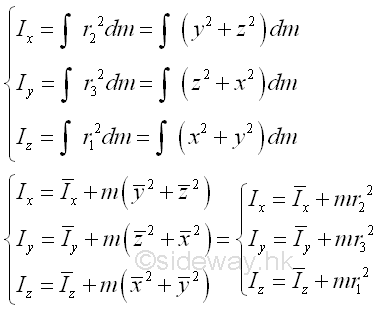Moment of Inertia of a Mass of Homogeneous Body

Moment of Inertia of Slender Rod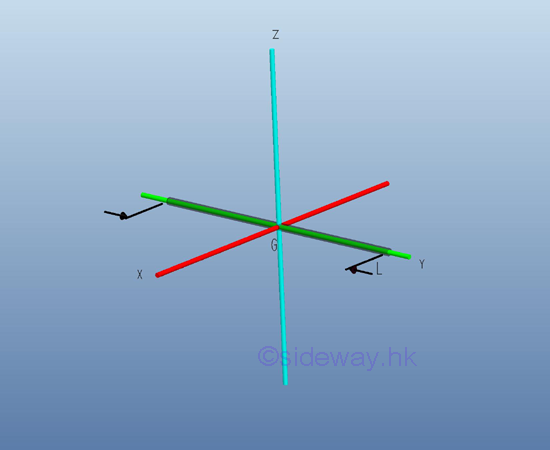Consider a slender homogenous circular rod of length L with uniform cross-sectional area A and homogenouse material density ρ. Both the cross-sectional area and the material density are constant over the lenght. Since the cross-sectional radius r is much smaller than the length of slender rod, the mass and the elemental mass of the slender rod can be expressed in terms of the mass per unit length, if the reference axis lies in centre of gravity of the slender rod, the mass moment of inertia of the slender rod with respect to an centroidal axis can be expressed as the elemental mass on the axial axis with the distance x as the radius between the elemental mass and the reference axis. Imply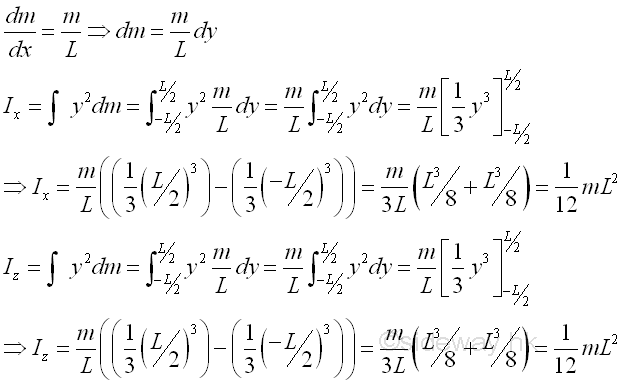Moment of Inertia of Circular CylinderConsider a homogenous circular cylinder of length L with uniform cross-sectional area A of radius r and homogenouse material density l. Both the cross-sectional area and the material density are constant over the lenght, the mass and the elemental mass of the circular cylinder can be expressed in terms of the volume of the circular cylinder. Since the cross-sectional radius r cannot be neglected, the actual distance between the elemental mass and the reference axis should be used. The moments of inertia about axes x and z, can be determined by parallel-axis theorem. Imply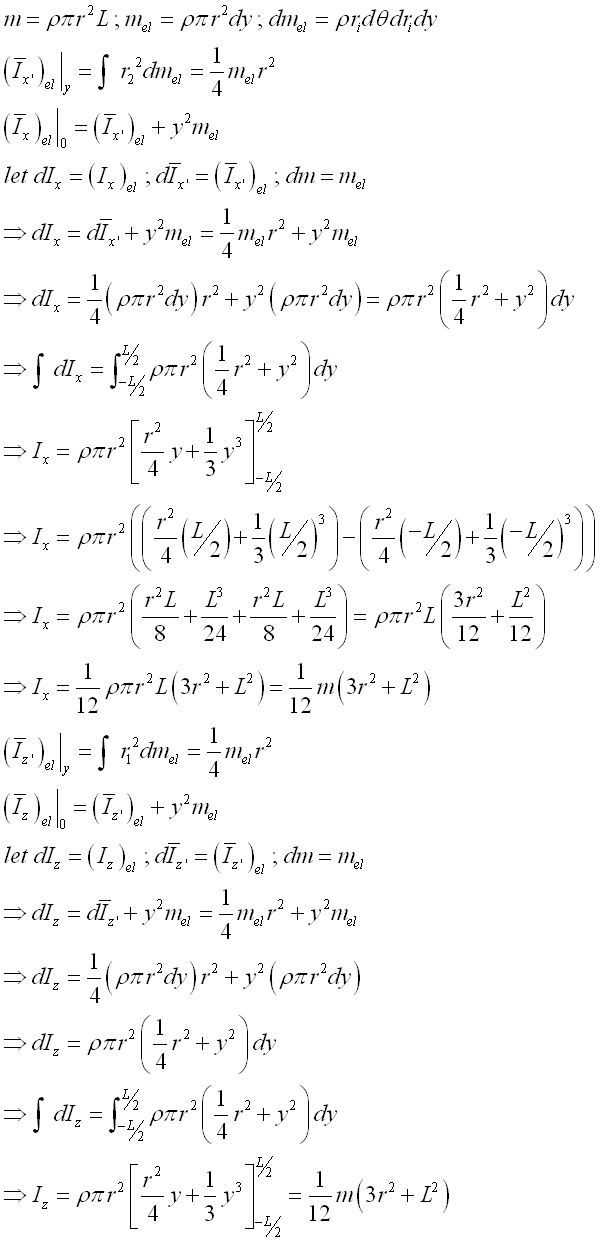And the moments of inertia about axis y can also be determined by parallel-axis theorem. Imply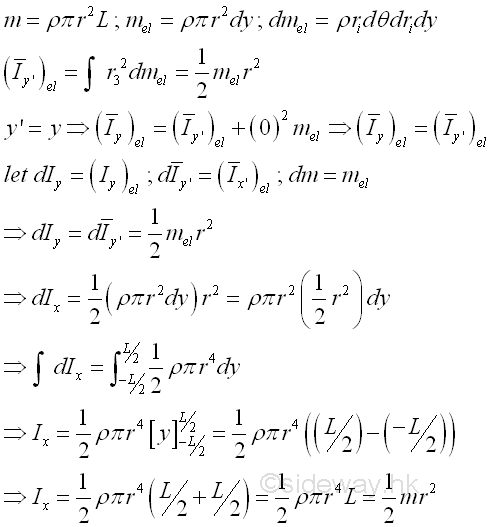Moment of Inertia of Rectangular PrismConsider a homogenous rectangular prism of length L with uniform cross-sectional area A of height h, width b and homogenouse material density l. Both the cross-sectional area and the material density are constant over the lenght, the mass and the elemental mass of the rectangular prism can be expressed in terms of the volume of the rectangular prism. Since the cross-sectional dimensions cannot be neglected, the actual distance between the elemental mass and the reference axis should be used. The moments of inertia about axes x and z, can be determined by parallel-axis theorem. ImplyAnd the moments of inertia about axis y can also be determined by parallel-axis theorem. ImplyMoment of Inertia of Circular Cone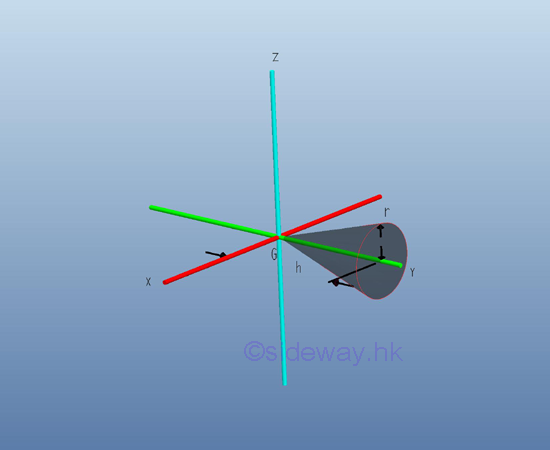Consider a homogenous circular cone of height h with base area A of radius r and homogenouse material density l. The material density is a constant, the mass and the elemental mass of the circular cone can be expressed in terms of the volume of the circular cone. Since the radius r of the base area cannot be neglected, the actual distance between the elemental mass and the reference axis should be used. The moments of inertia about axes x and z, can be determined by parallel-axis theorem. Imply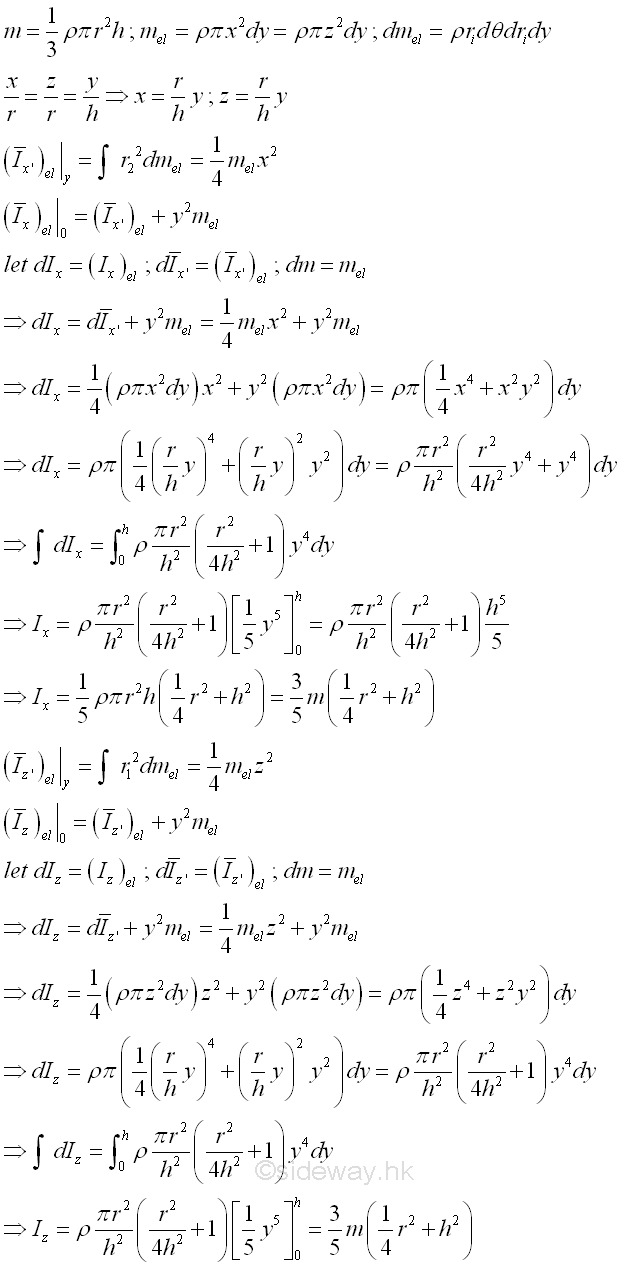And the moments of inertia about axis y can also be determined by parallel-axis theorem. Imply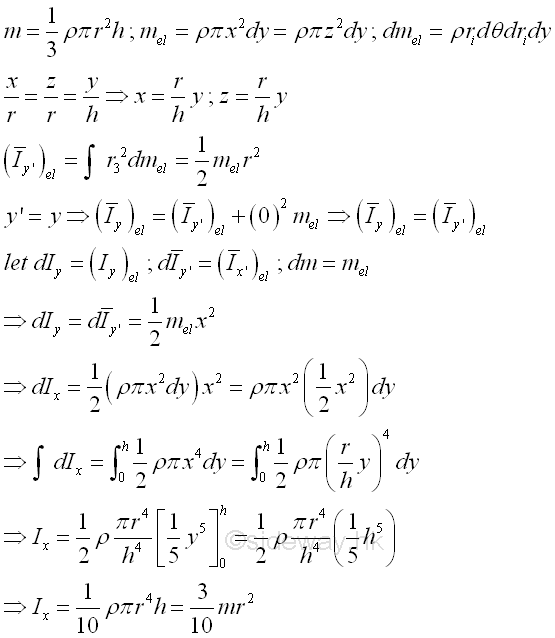Moment of Inertia of Sphere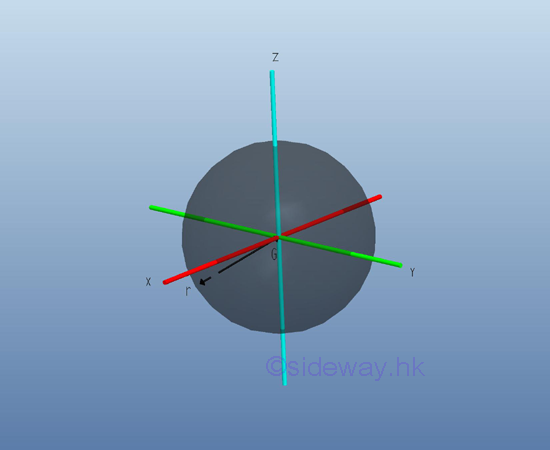Consider a homogenous sphere of radius r and homogenouse material density l. The material density is a constant, the mass and the elemental mass of the circular cone can be expressed in terms of the volume of the circular cone. Since the radius r of the sphere area cannot be neglected, the actual distance between the elemental mass and the reference axis should be used. The moments of inertia about axes x, y and z, can be determined by triple integration. Imply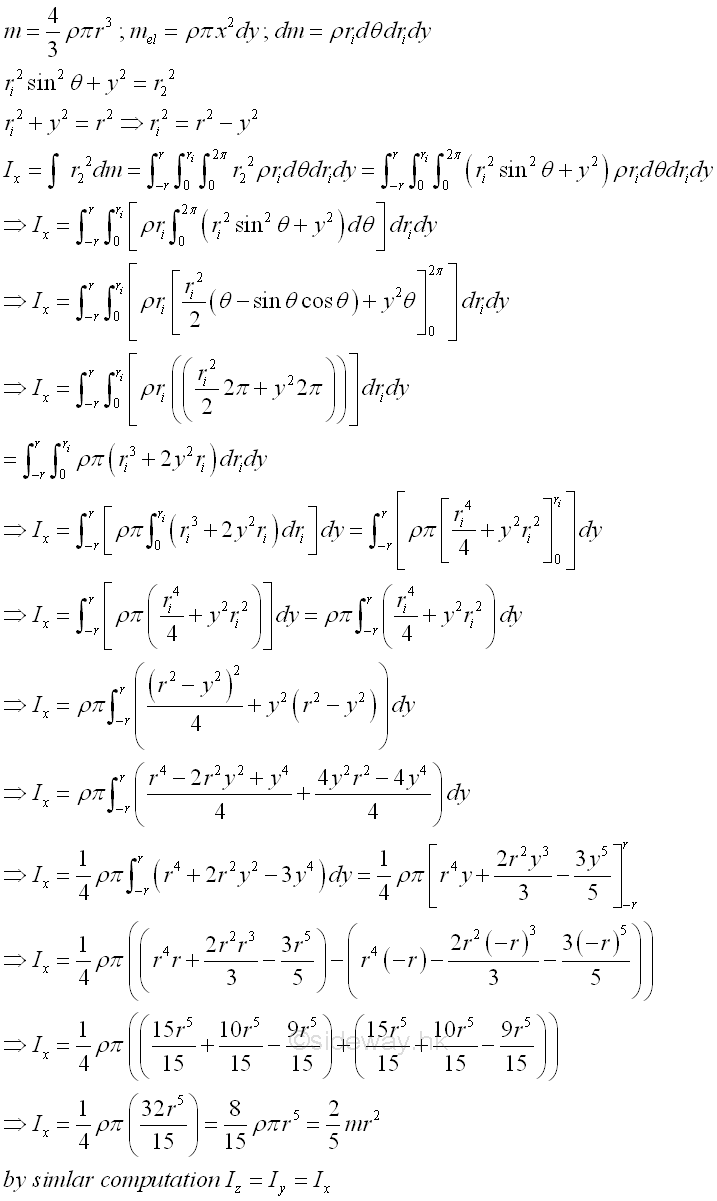References

1. I.C. Jong; B.G. rogers, 1991, Engineering Mechanics: Statics and Dynamics, Saunders College Publishing, United States of America
2. F.P. Beer; E.R. Johnston,Jr.; E.R. Eisenberg, 2004, Vector Mechanics for Engineers: Statics, McGraw-Hill Companies, Inc., New YorkID: 121100086 Last Updated: 2012/11/14 Revision: 0 Ref:Home (5)

Management

HBR (3)

Information

Recreation

Hobbies (7)

Culture

Chinese (1097)

English (336)

Reference (66)

Computer

Hardware (149)

Software

Application (187)

Digitization (24)

Numeric (19)

Programming

Web (704)CSS (SC)

ASP.NET (SC)

HTML

Knowledge Base

Common Color (SC)

Html 401 Special (SC)

OS (389)

MS Windows

Windows10 (SC)

.NET Framework (SC)

DeskTop (7)

Knowledge

Mathematics

Formulas (8)

Algebra (20)

Number Theory (206)

Trigonometry (18)

Geometry (18)

Calculus (67)

Complex Analysis (21)

Engineering

Tables (8)

Mechanical

Mechanics (1)

Rigid Bodies

Statics (92)

Dynamics (37)

Fluid (5)

Control

Acoustics (19)

Biology (1)

Geography (1)